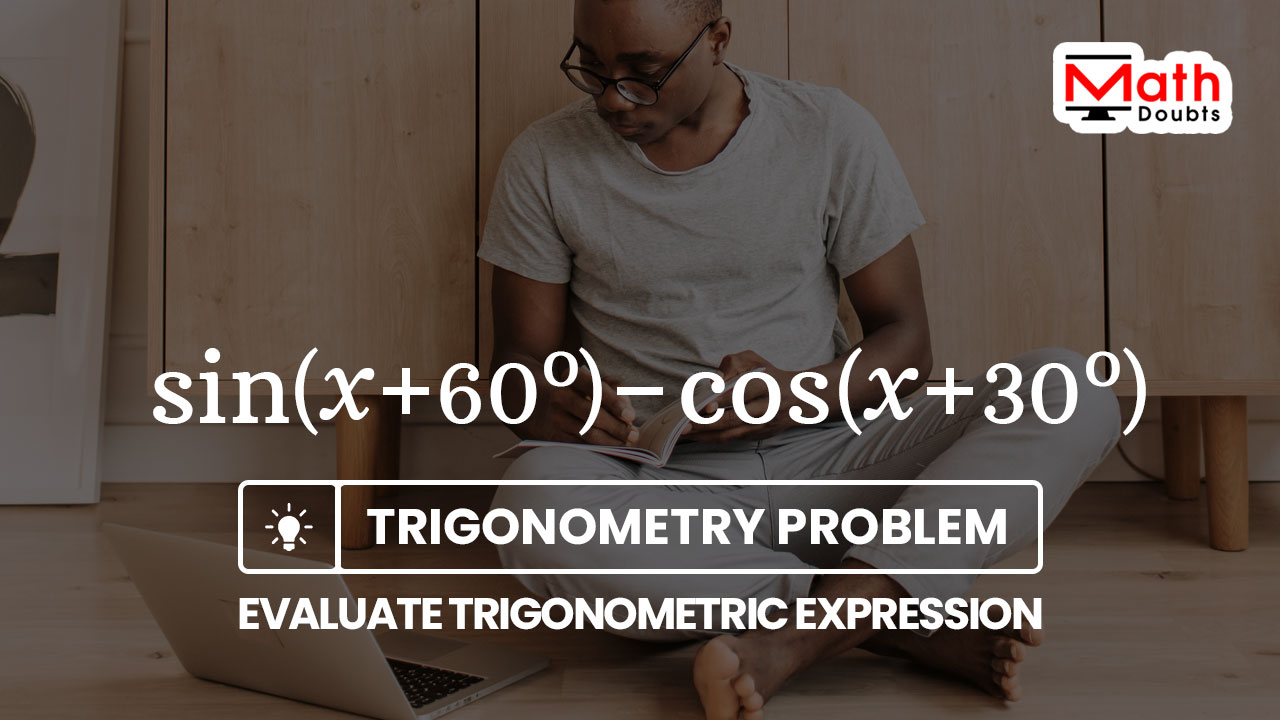# Evaluate $\sin{(x+60^\circ)}$ $-$ $\cos{(x+30^\circ)}$

The value of the cosine of angle $x$ plus thirty degrees should be subtracted from the value of the sine of angle $x$ plus sixty degrees to find its value in this trigonometry problem.In trigonometry, there is no trigonometric identity to subtract the cosine function from the sine function but the angles inside the trigonometric functions are compound angles. So, let’s learn how to simplify the sine of angle $x$ plus sixty degrees minus cosine of angle $x$ plus thirty degrees to find its value.

### Expand the sine of sum of two angles

In the first term, the angles $x$ and sixty degrees are connected by a plus sign to form a compound angle inside the sine function. The angle sixty degrees is a standard angle in trigonometry and the values of trigonometric functions of angle $60^\circ$ are known to us. So, it is a good idea to expand the sine of the sum of two angles $x$ and $60^\circ$ by the angle sum identity of sine function.

$\implies$ $\sin{(x+60^\circ)}$ $\,=\,$ $\sin{x}\cos{(60^\circ)}$ $+$ $\sin{x}\cos{(60^\circ)}$

$\,\,=\,\,$ $\sin{x} \times \cos{(60^\circ)}$ $+$ $\cos{x} \times \sin{(60^\circ)}$

According to the trigonometry, the exact value of cosine of sixty degrees is one divided by two and the exact value of sine of sixty degrees is the square root of three divided by two.

$\,\,=\,\,$ $\sin{x} \times \dfrac{1}{2}$ $+$ $\cos{x} \times \dfrac{\sqrt{3}}{2}$

A trigonometric function is multiplied by a fraction in each term of the trigonometric expression and the products of them can be evaluated by the multiplication of the fractions.

$\,\,=\,\,$ $\dfrac{\sin{x}}{1} \times \dfrac{1}{2}$ $+$ $\dfrac{\cos{x}}{1} \times \dfrac{\sqrt{3}}{2}$

$\,\,=\,\,$ $\dfrac{\sin{x} \times 1}{1 \times 2}$ $+$ $\dfrac{\cos{x} \times \sqrt{3}}{1 \times 2}$

$\,\,=\,\,$ $\dfrac{\sin{x} \times 1}{2}$ $+$ $\dfrac{\cos{x} \times \sqrt{3}}{2}$

The places of the factors in the numerator of both terms in the trigonometric expression can be changed for our convenience by using the commutative property of the multiplication.

$\,\,=\,\,$ $\dfrac{1 \times \sin{x}}{2}$ $+$ $\dfrac{\sqrt{3} \times \cos{x}}{2}$

$\,\,\,\therefore\,\,\,\,\,\,$ $\sin{(x+60^\circ)}$ $\,=\,$ $\dfrac{\sin{x}}{2}$ $+$ $\dfrac{\sqrt{3}\cos{x}}{2}$

### Expand the cosine of sum of two angles

In the second term, the angles $x$ and thirty degrees are connected by a plus sign to form a compound angle inside the cosine function. The angle $30^\circ$ is also a standard angle and the exact values of the trigonometric functions of angle thirty degrees are known to us. So, let’s expand the cosine of the sum of two angles $x$ and $30^\circ$ by the angle sum identity of cosine function.

$\implies$ $\cos{(x+30^\circ)}$ $\,=\,$ $\cos{x}\cos{(30^\circ)}$ $-$ $\sin{x}\sin{(30^\circ)}$

$\,\,=\,\,$ $\cos{x} \times \cos{(30^\circ)}$ $-$ $\sin{x} \times \sin{(30^\circ)}$

According to the trigonometry, the exact value of cos of thirty degrees is equal to the square root of three divided by two and the exact value of sin of thirty degrees is equal to one divided by two.

$\,\,=\,\,$ $\cos{x} \times \dfrac{\sqrt{3}}{2}$ $-$ $\sin{x} \times \dfrac{1}{2}$

In the trigonometric expression, a trigonometric function is multiplied by a fraction in each term and the products of them can be evaluated by using the multiplication of the fractions.

$\,\,=\,\,$ $\dfrac{\cos{x}}{1} \times \dfrac{\sqrt{3}}{2}$ $-$ $\dfrac{\sin{x}}{1} \times \dfrac{1}{2}$

$\,\,=\,\,$ $\dfrac{\cos{x} \times \sqrt{3}}{1 \times 2}$ $-$ $\dfrac{\sin{x} \times 1}{1 \times 2}$

In each term, the places of factors can be changed in the numerator of the trigonometric expression by using the commutative property of the multiplication.

$\,\,=\,\,$ $\dfrac{\sqrt{3} \times \cos{x}}{2}$ $-$ $\dfrac{1 \times \sin{x}}{2}$

$\,\,\, \therefore \,\,\,\,\,\, \cos{(x+30^\circ)}$ $\,=\,$ $\dfrac{\sqrt{3}\cos{x}}{2}$ $-$ $\dfrac{\sin{x}}{2}$

### Evaluate the expression by simplification

In the above two steps, the sine and cosine of compound angles are expanded by using the angle sum trigonometric identities.

$(1).\,\,$ $\sin{(x+60^\circ)}$ $\,=\,$ $\dfrac{\sin{x}}{2}$ $+$ $\dfrac{\sqrt{3}\cos{x}}{2}$

$(2).\,\,$ $\cos{(x+30^\circ)}$ $\,=\,$ $\dfrac{\sqrt{3}\cos{x}}{2}$ $-$ $\dfrac{\sin{x}}{2}$

Now, let’s substitute them in the given trigonometric expression to find its value by simplifying the trigonometric expression.

$\implies$ $\sin{(x+60^\circ)}$ $-$ $\cos{(x+30^\circ)}$ $\,=\,$ $\dfrac{\sin{x}}{2}$ $+$ $\dfrac{\sqrt{3}\cos{x}}{2}$ $-$ $\bigg(\dfrac{\sqrt{3}\cos{x}}{2}$ $-$ $\dfrac{\sin{x}}{2}\bigg)$

The trigonometric expression on the right hand side of the equation should be simplified to find the value of the given trigonometric expression. So, let’s concentrate on simplifying the trigonometric expression on the right hand side of the equation.

$\,\,=\,\,$ $\dfrac{\sin{x}}{2}$ $+$ $\dfrac{\sqrt{3}\cos{x}}{2}$ $-$ $\dfrac{\sqrt{3}\cos{x}}{2}$ $+$ $\dfrac{\sin{x}}{2}$

The second and third terms in the trigonometric expression are same but they have opposite signs. So, they both get cancelled each other in the trigonometric expression.

$\,\,=\,\,$ $\dfrac{\sin{x}}{2}$ $+$ $\cancel{\dfrac{\sqrt{3}\cos{x}}{2}}$ $-$ $\cancel{\dfrac{\sqrt{3}\cos{x}}{2}}$ $+$ $\dfrac{\sin{x}}{2}$

$\,\,=\,\,$ $\dfrac{\sin{x}}{2}$ $+$ $\dfrac{\sin{x}}{2}$

The two terms are fractions and they both have same denominators. So, the two fractions can be added by the addition of the like fractions.

$\,\,=\,\,$ $\dfrac{\sin{x}+\sin{x}}{2}$

$\,\,=\,\,$ $\dfrac{2\sin{x}}{2}$

$\,\,=\,\,$ $\dfrac{2 \times \sin{x}}{2}$

$\,\,=\,\,$ $\dfrac{\cancel{2} \times \sin{x}}{\cancel{2}}$

$\,\,=\,\,$ $\sin{x}$

Latest Math Topics
Jun 26, 2023
Jun 23, 2023

Latest Math Problems
Jul 01, 2023
Jun 25, 2023
###### Math Questions

The math problems with solutions to learn how to solve a problem.

Learn solutions

Practice now

###### Math Videos

The math videos tutorials with visual graphics to learn every concept.

Watch now

###### Subscribe us

Get the latest math updates from the Math Doubts by subscribing us.